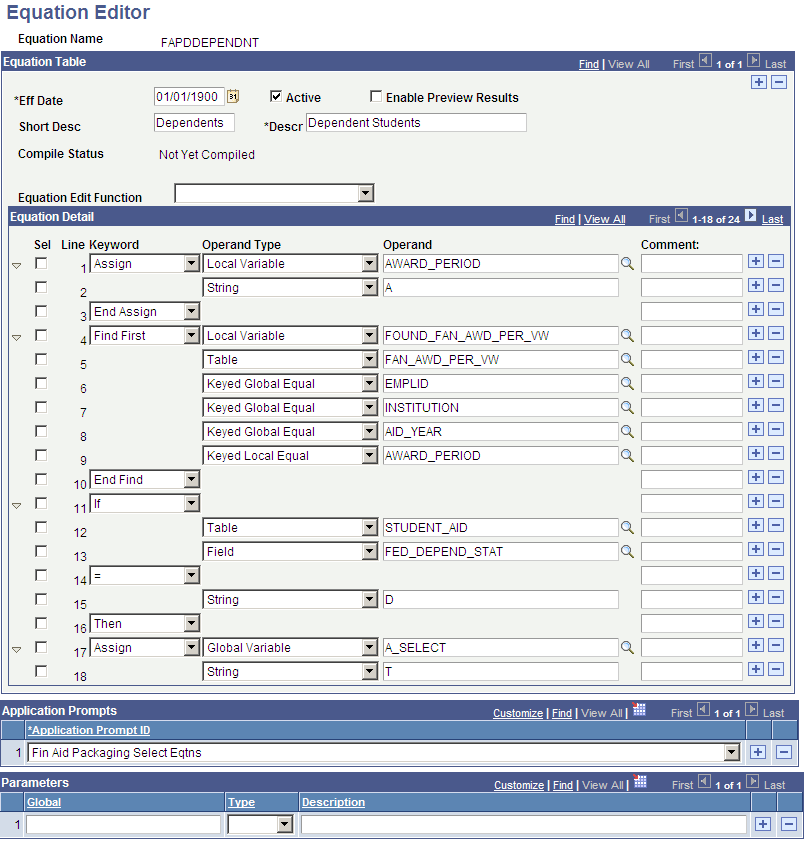Defining an Equation

This section provides an overview of application prompts, lists a prerequisite, and discusses how to define equations and application prompts.

If you are working on a particular feature, such as a packaging plan, and need to create one or more equations for that plan, you must define those equations here and include a short description and long description for the new equation.

You must then associate that equation with an application prompt. For example, the equation that you are creating is a packaging selection equation. Because this equation will be used to access a population of students—that is, it is a selection equation—you must assign the Fin Aid Packaging Enter Eqtn application prompt to the equation. You do that by selecting Fin Aid Packaging Select Eqtn from the drop-down list box. By associating the application prompt value with an equation here, this equation will appear in the list of available selection equations that you can use when creating your packaging plan in the Packaging Plan Setup table.

As another example, if you are working on a Student Financials tuition calculation feature, and you want to create an equation that will return an amount, you must associate your new equation with an application prompt. You would select Stdnt Fin Tuition Amount Eqtns or Stdn Fin Tuit Amount Limited. After selecting one of these application prompts here, your new equation will appear on the list of possible equations to use when working with the tuition calculation setup feature.

Warning! Do not make any changes to the Application Prompt values.

The application prompts Stdnt Fin Tuit Select Limited and Stdnt Fin Tuit Amount Limited are used by equations that use only global variables from the STDNT_CAR_TERM record. Both prompts eliminate the need to repeatedly call the Equation Engine.

This table lists the application prompt to use based on the global variable:

Student Financials Global Variables

Stdnt Fin Tuition Amount Eqtns

Stdnt Fin Tuition Select Eqtns

Stdnt Fin Tuition Amount Limited

Stdnt Fin Tuit Select Limited

INSTITUTION

X

X

X

X

X

X

X

X

X

X

X

X

STRM (term)

X

X

X

X

EMPLID

X

X

X

X

STDNT_CAR_NBR (student career number)

X

X

X

X

BILLING_CAREER

X

X

X

X

A_AMOUNT

X

X

X

X

A_SELECT (yes/no check box)

X

X

X

X

SESSION_CODE

X

X

NA

NA

CLASS_NBR (class number)

X

X

NA

NA

CRSE_ID

(course ID)

X

X

NA

NA

TRANS_DATE (enrl_drop_dt)

X

X

NA

NA

ENRL_ACTN_RSN_LAST (enrolment action reason last)

X

X

NA

NA

TRANS_TIME (last drop term stamp)

X

X

NA

NA

Note: NA = Not Applicable

Before you define an equation on the Equation Editor page, you should devise a flow chart that considers what your end result should be. Using a flow chart will help you develop proper equation syntax.

Page Name

Definition Name

Usage

Equation Editor

EQUATION_EDITOR

select Set Up SACR, then select Common Definitions, then select Equation Engine, then select Equation Editor

Define an equation and application prompts.

Access the Equation Editor page (select Set Up SACR, then select Common Definitions, then select Equation Engine, then select Equation Editor).

Image: Equation Editor (FAPDDEPENDNT) page

This example illustrates the fields and controls on the Equation Editor (FAPDDEPENDNT) page. You can find definitions for the fields and controls later on this page.Equation Table

Field or Control

Definition

Eff Date(effective date)

Enter a date after which the equation will be effective.

Active

This check box is selected when an equation is available for use.

Enable Preview Results

Select to preview results based on the parameters that you selected before you run the process. This check box can be selected on the Selection Tool page and is associated with the Pop Select feature.

Short Desc(short description)

Enter a short description for the equation based on the equation naming guidelines.

Descr(description)

Enter a description for this equation.

Equation Edit Function

Select an equation edit function value. This drop-down list box contains a selection of predefined edit functions to assist with tasks such as cutting and pasting, compiling, and printing an equation.

Equation Detail

Field or Control

Definition

Keyword

Select equation keywords that are necessary for your equation.

Operand Type

Select an operand type from the available options. This operand identifies the type of information contained in the operand that follows.

Operand

Enter the operand based on the operand type selected in the previous field.

Comment

Enter a short comment. This is not part of the equation.

Application Prompts

Field or Control

Definition

Application Prompt ID

Select an application prompt value. These prompt values control which equations will be available for you to choose from when defining equations for a particular feature. If you do not select an application prompt value for an equation here, that equation will not appear on the list of available equations for selection during feature processing.

Prompt values include:

Fin Aid Loan Edits for CL4

Fin Aid Loan Edits for CRC

Fin Aid Packaging Amount Eqtns

Fin Aid Packaging Select Eqtns

Stdnt Fin Tuition Amount Eqtns

Stdnt Fin Tuition Selct Eqtns

Stdnt Fin Tuit Select Limited

Stdnt Fin Tuit Amount Limited

Parameters

Field or Control

Definition

Global

Enter a global variable value.

Type

Select an operand type from the available options. The operand type identifies the type of information contained in the operand that follows.